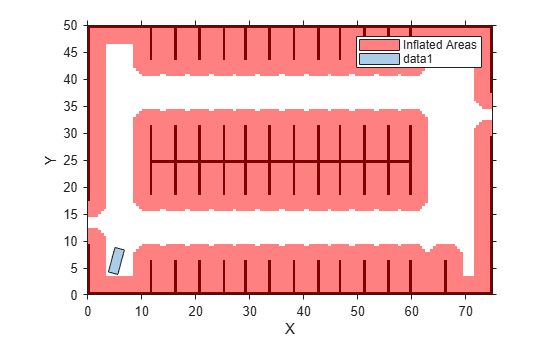# plot

Plot vehicle costmap

## Syntax

``plot(costmap)``
``plot(costmap,Name,Value)``

## Description

The `plot` function displays a vehicle costmap. The darkness of each cell is proportional to the cost value of the cell. Cells with low cost are bright, and cells containing obstacles with high cost are dark. Inflated areas are displayed with a red hue, and cells outside the inflated area are displayed in grayscale.

example

````plot(costmap)` plots the vehicle costmap in the current axes.```
````plot(costmap,Name,Value)` plots the vehicle costmap using name-value pair arguments to specify the parent axes or to adjust the display of inflated areas.```

## Examples

collapse all

Load a costmap from a parking lot. Display the costmap.

```data = load('parkingLotCostmap.mat'); parkMap = data.parkingLotCostmap; plot(parkMap)```

Create a template polyshape object with the dimensions of the car.

`carDims = parkMap.CollisionChecker.VehicleDimensions`
```carDims = vehicleDimensions with properties: Length: 4.7000 Width: 1.8000 Height: 1.4000 Wheelbase: 2.8000 RearOverhang: 1 FrontOverhang: 0.9000 WorldUnits: 'meters' ```
```ro = carDims.RearOverhang; fo = carDims.FrontOverhang; wb = carDims.Wheelbase; hw = carDims.Width/2; X = [-ro,wb+fo,wb+fo,-ro]; Y = [-hw,-hw,hw,hw]; templateShape = polyshape(X',Y');```

Create a function handle to move the template to a specified vehicle pose. This `move` function translates the polyshape `s` to the coordinate (`x`,`y`) and then rotates the polyshape by an angle `theta` about the point (`x`,`y`).

```move = @(s,x,y,theta) rotate(translate(s,[x,y]), ... theta,[x,y]);```

Move the car template to a pose.

```carPose = [5,5,75]; carShape = move(templateShape,carPose(1),carPose(2),carPose(3));```

Plot the car on the costmap.

```hold on plot(carShape)```## Input Arguments

collapse all

Costmap, specified as a `vehicleCostmap` object.

### Name-Value Arguments

Specify optional pairs of arguments as `Name1=Value1,...,NameN=ValueN`, where `Name` is the argument name and `Value` is the corresponding value. Name-value arguments must appear after other arguments, but the order of the pairs does not matter.

Before R2021a, use commas to separate each name and value, and enclose `Name` in quotes.

Example: `'Inflation','off'`

Display inflated areas, specified as the comma-separated pair consisting of `'Inflation'` and one of the following.

• `'on'`—Cells in the inflated area have a red hue.

• `'off'`—Cells containing obstacles have a red hue, but other cells in the inflated area are displayed in grayscale.

Axes on which to plot the costmap, specified as the comma-separated pair consisting of `'Parent'` and an axes handle. By default, `plot` uses the current axes handle, which is returned by the `gca` function.

## Version History

Introduced in R2018a